# Hypergraph

(diff) ← Older revision | Latest revision (diff) | Newer revision → (diff)

A generalization of the concept of a graph. A hypergraph is defined by a set, whose elements are known as vertices, and by a familyof subsets of, known as edges or hyperedges. A hypergraph is denoted by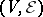. The concept of a hypergraph is a variant of the familiar concepts of a complex, a block design and a network.

Two vertices of a hypergraph are said to be adjacent if there exists an edge containing these vertices. A vertexand an edgeof a hypergraph are said to be incident if. A hypergraphwithvertices andedges may be defined by an incidence matrix, i.e. by the matrix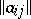of dimension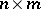in which the columns correspond to the edges while the rows correspond to the vertices of the hypergraph, and whereIt is possible to assign to each matrixconsisting of zeros and ones a hypergraph for whichis the incidence matrix. The hypergraph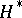is called the dual of the hypergraphif the incidence matrix ofis obtained by transposing the incidence matrix of. The number of edges of a hypergraphthat are incident to a given vertex is called the degree of the vertex. The degree of an edge is the number of vertices of the hypergraph incident to this edge. A hypergraph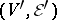is called a subhypergraph of a hypergraphif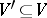,, and if a vertexfrom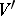and an edgefromare incident in the hypergraphif and only if they are incident in the hypergraph.

A hypergraph may be represented in a plane by identifying the vertices of the hypergraph with points of the plane and by identifying the edges with connected domains containing the vertices incident with these edges. For instance, it is possible to represent the hypergraphwith set of vertices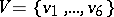and family of edgesin a plane, as shown in the figure.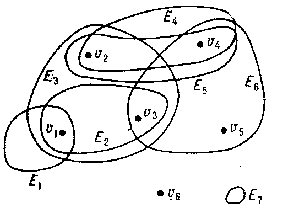Figure: h048470a

A hypergraphmay be represented by a bipartite graph (cf. Graph, bipartite)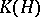in which the vertices of one partcorrespond to the vertices of the hypergraph, while the vertices of the other part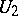correspond to the edges of. Two vertices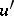from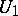andfromwill be connected by an edge in the graphif the vertex of the hypergraph corresponding tois incident to the edge of the hypergraph corresponding to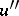. A hypergraph is a graph if each of its edges has degree two. An important special case of the concept of a "hypergraph" is that of a matroid. Many concepts in the theory of graphs, such as connectedness, planarity, the chromatic number, and the external and internal stability numbers, may be applied to hypergraphs, as may many theorems that are applicable to graphs.

How to Cite This Entry:
Hypergraph. Encyclopedia of Mathematics. URL: http://encyclopediaofmath.org/index.php?title=Hypergraph&oldid=11526
This article was adapted from an original article by A.A. Sapozhenko (originator), which appeared in Encyclopedia of Mathematics - ISBN 1402006098. See original article